You are here: Start » AVL.NET » Geometry 2D Features

# Geometry 2D Features

Icon Name Description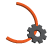ArcCircle Computes the circle containing an arc.ArcEndpoints Computes two endpoints of an arc.ArcLength Computes the length of an arc.ArcMidpoint Computes the midpoint of an arc.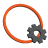CircleArea Computes the area of a circle.CircleBoundingBox Computes the smallest box containing a circle.CircleBoundingRectangle Computes the smallest rectangle containing a circle.CircleCharacteristicPoint Returns a characteristic point (e.g. the top-left) of a box containing the input circle.CirclePerimeterLength Computes the length of a circle perimeter.CircleSection Computes an arciform section of the circle perimeter.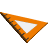LineNormalVector Computes the normal vector of a lineLineOrientation Computes the orientation angle of a line as angle in the range from 0 to 180.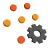PointsBoundingBox Computes the smallest box containing an array of points.PointsBoundingBox_OrNil Computes the smallest box containing an array of points; returns NIL if the array is empty.PointsBoundingCircle Computes the smallest circle containing an array of points.PointsBoundingCircle_OrNil Computes the smallest circle containing an array of points; returns NIL if the array is empty.PointsBoundingParallelogram Computes the smallest parallelogram containing an array of points.PointsBoundingRectangle Computes the smallest rectangle containing an array of points.PointsBoundingRectangle_FixedAngle Computes the smallest rectangle with the given orientation angle containing an array of points.PointsBoundingRectangle_FixedAngle_OrNil Computes the smallest rectangle with the given orientation angle containing an array of points; returns NIL when the array is empty.PointsBoundingRectangle_OrNil Computes the smallest rectangle containing an array of points; returns NIL when the array is empty.PointsCaliperDiameter Computes the longest and the shortest width of the input points measured as distance between parallel lines containing all of them.PointsConvexHull Computes the smallest convex shape that contains the given array of points.PointsDiameter Finds the longest segment connecting two points from a given array.PointsMassCenter Computes the central point of the input points.PointsMassCenter_OrNil Computes the central point of the input points; returns NIL if the array is empty.PointsMedian Computes the geometric median of the input points.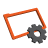RectangleArea Computes the area of a rectangle.RectangleBoundingBox Computes the smallest box containing a rectangle.RectangleBoundingCircle Computes the smallest circle containing a rectangle.RectangleCenter Computes the center point of a rectangle.RectangleCharacteristicPoint Returns a characteristic point (e.g. the top-left) of the input rectangle.RectangleCharacteristicPoints Computes rectangle's characteristic points.RectangleCorners Computes the four corner points of a rectangle.RectanglePerimeterLength Computes the perimeter length of a rectangle.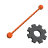SegmentBisector Computes a line passing through the center of a segment at a right angle.SegmentCenter Computes the center point of a segment.SegmentLength Computes the length of a segment.SegmentLine Computes the line passing through a segment.SegmentNormalVector Computes an unitary vector perpendicular to the segment.SegmentOrientation Computes the orientation of a segment.SegmentVector Returns the vector [x2 - x1, y2 - y1]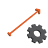VectorDirection Computes the direction angle of a vector as an angle in range the from 0 to 360.VectorLength Computes the length of a vector.VectorsMedian Computes the geometric median of the input vectors.